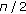# The Best Approximation of the Sinc Function by a Polynomial of Degreewith the Square Norm

## Abstract

The polynomial of degree n which is the best approximation of the sinc function on the interval (0,] with the square norm is considered. By using Lagrange's method of multipliers, we construct the polynomial explicitly. This method is also generalized to the continuous function on the closed interval [a, b]. Numerical examples are given to show the effectiveness.

## Author information

Authors

### Corresponding author

Correspondence to Yuyang Qiu.

## Rights and permissions

Reprints and Permissions

Qiu, Y., Zhu, L. The Best Approximation of the Sinc Function by a Polynomial of Degreewith the Square Norm. J Inequal Appl 2010, 307892 (2010). https://doi.org/10.1155/2010/307892

• Accepted:

• Published:

• DOI: https://doi.org/10.1155/2010/307892

### Keywords

• Continuous Function
• Closed Interval